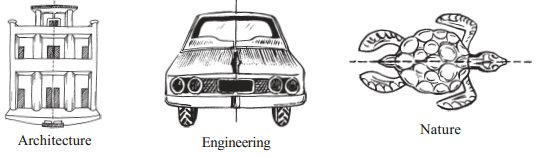# Symmetry worksheet for class 7## myCBSEguide App

CBSE, NCERT, JEE Main, NEET-UG, NDA, Exam Papers, Question Bank, NCERT Solutions, Exemplars, Revision Notes, Free Videos, MCQ Tests & more.

## Symmetry worksheet for class 7 Important Topics

• Recalling reflection symmetry
• Line Symmetry
• Rotational Symmetry
• A comparison between line symmetry and rotational symmetry## Some important Facts about Symmetry worksheet for class 7

1. Name any two figures that have both line symmetry and rotational symmetry.
2. Draw, wherever possible, a rough sketch of
1. a triangle with both line and rotational symmetries of order more than 1.
2. a triangle with only line symmetry and no rotational symmetry of order more than 1.
3. a quadrilateral with a rotational symmetry of order more than 1 but not a line symmetry.
4. a quadrilateral with line symmetry but not a rotational symmetry of order more than 1.
3. If a figure has two or more lines of symmetry, should it have rotational symmetry of order more than 1?

## NCERT class 7Mathematics Solved Worksheets

• Chapter 1 – Integers
• Chapter 2 – Fractions and Decimals
• Chapter 3 – Data Handling
• Chapter 4 – Simple Equations
• Chapter 5 – Lines and Angles
• Chapter 6 – Practical Geometry
• Chapter 7 – The Triangle and its Properties
• Chapter 8 – Congruence of triangles
• Chapter 9 – Comparing Quantities
• Chapter 10 – Rational numbers
• Chapter 11 – Perimeter and Area
• Chapter 12 – Algebraic Expressions
• Chapter 13 – Exponents and Powers
• Chapter 14 – Symmetry
• Chapter 15 – Visualizing Solid Shapes

## LINES OF SYMMETRY FOR REGULAR POLYGONS

You know that a polygon is a closed figure made of several line segments. The polygon made up of the least number of line segments is the triangle. (Can there be a polygon that you can draw with still fewer line segments? Think about it).

A polygon is said to be regular if all its sides are of equal length and all its angles are of equal measure. Thus, an equilateral triangle is a regular polygon of three sides. Can you name the regular polygon of four sides?00 kg. These numbers are not convenient to write and read. To make it convenient we use powers.

To download Printable worksheets for class 7 Mathematics and Science; do check myCBSEguide app or website. myCBSEguide provides sample papers with solution, test papers for chapter-wise practice, NCERT solutions, NCERT Exemplar solutions, quick revision notes for ready reference, CBSE guess papers and CBSE important question papers. Sample Paper all are made available through the best app for CBSE students and myCBSEguide website.